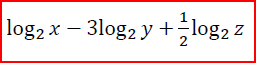Ch.3: Pre-Calc (Part 3)WorksheetSee all chapters

# Logarithmic Functions

See all sections
Sections
Inverse Functions
Exponential Functions
Logarithmic Functions

Concept #1: Log Graphs, Rules of Logarithms

Concept #2: Intro

Practice: Expand the following logarithm.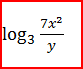Practice: Expand the following logarithm.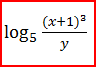Practice: Expand the following logarithm.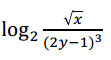Practice: Compress the following logarithm.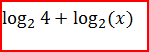Practice: Compress the following logarithm.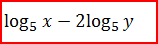Practice: Compress the following logarithm.